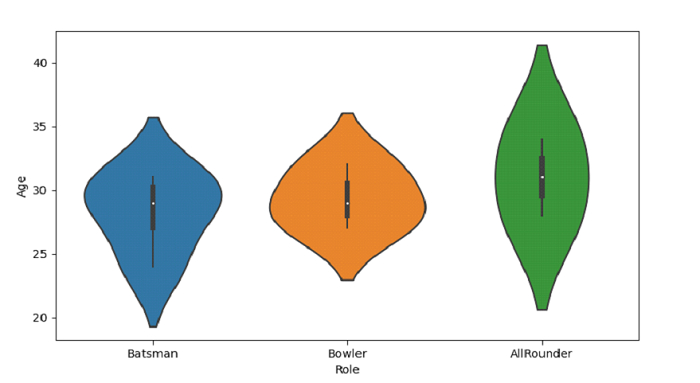# Python Pandas - Draw a vertical violinplot grouped by a categorical variable with Seaborn

PythonServer Side ProgrammingProgramming

#### Beyond Basic Programming - Intermediate Python

Most Popular

36 Lectures 3 hours

#### Practical Machine Learning using Python

Best Seller

91 Lectures 23.5 hours

#### Practical Data Science using Python

22 Lectures 6 hours

Violin Plot in Seaborn is used to draw a combination of boxplot and kernel density estimate. The seaborn.violinplot() is used for this. We will plotti violin plot with the columns grouped by a categorical variable.

Let’s say the following is our dataset in the form of a CSV file − Cricketers.csv

At first, import the required libraries −

import seaborn as sb
import pandas as pd
import matplotlib.pyplot as plt

Load data from a CSV file into a Pandas DataFrame −

dataFrame = pd.read_csv("C:\Users\amit_\Desktop\Cricketers.csv")


Plotting violin plot with Role and Age grouped by a categorical variable −

sb.violinplot(x = 'Role', y = "Age", data = dataFrame)

## Example

Following is the code −

import seaborn as sb
import pandas as pd
import matplotlib.pyplot as plt

# Load data from a CSV file into a Pandas DataFrame:

# plotting violin plot with Role and Age
# grouped by a categorical variable
sb.violinplot(x = 'Role', y = "Age", data = dataFrame)

# display
plt.show()

## Output

This will produce the following output −